# Mean field models

A mean field model, or a mean field solution of a model, is an approximation to the actual solution of a model in statistical physics. The model is made exactly solvable by treating the effect of all other particles on a given one as a mean field (hence its name). It appear in different forms and different contexts, but all mean field models have this feature in common.

## Mean field solution of the Ising model

A well-known mean field solution of the Ising model, known as the Bragg-Williams approximation goes as follows. From the original Hamiltonian,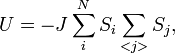$U = - J \sum_i^N S_i \sum_{} S_j ,$

suppose we may approximate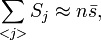$\sum_{} S_j \approx n \bar{s},$

where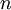$n$ is the number of neighbors of site$i$ (e.g. 4 in a 2-D square lattice), and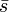$\bar{s}$ is the (unknown) magnetization: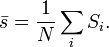$\bar{s}=\frac{1}{N} \sum_i S_i .$

Therefore, the Hamiltonian turns to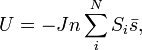$U = - J n \sum_i^N S_i \bar{s} ,$

as in the regular Langevin theory of magnetism: the spins are independent, but coupled to a constant field of strength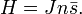$H= J n \bar{s}.$

The magnetization of the Langevin theory is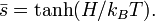$\bar{s} = \tanh( H/k_B T ).$

Therefore: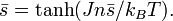$\bar{s} = \tanh(J n\bar{s}/k_B T).$

This is a self-consistent expression for$\bar{s}$. There exists a critical temperature, defined by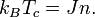$k_B T_c= J n .$

At temperatures higher than this value the only solution is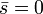$\bar{s}=0$. Below it, however, this solution becomes unstable (it corresponds to a maximum in energy), whereas two others are stable. Slightly below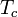$T_c$,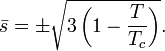$\bar{s} =\pm\sqrt{3\left(1 - \frac{T}{T_c}\right)}.$